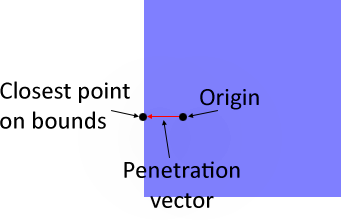# Simple AABB Collision Detection Using the Minkowski Difference (2014-10-04)

Since I’ve started on an adventure to start creating my games with Haxe and OpenFL, I found myself in need of some collision detection. I don’t really need anything as fancy or extensive as Nape, and although the HxCollision library is a pretty solid Separating Axis Theorem implementation, it doesn’t deal with swept-collisions, which is a bit of an issue for games (without swept collisions, any lag spikes can easily cause objects to pass right through objects!).

With some simple requirements in mind, I started Googling, and came across a method of using Minkowski addition to detect collisions. It turns out this method is super fast and very easy to compute for axis-aligned bounding-boxes (AABBs), which is all I’m going to focus on for now. This point is also only going to focus on discrete detection (whether or not an AABB is penetrating another object, regardless of velocity). I’ll do another post on using this method with swept collisions when I have that sorted out.

When you first read about Minkowski sums and differences, it can become very confusing very quickly (even if you are adept at math!). Luckily for us, the Minkowksi difference for two AABBs is itself an AABB, calculated as follows (assuming positive y is towards the bottom of the screen):

This tells us that when you compute the Minkowski difference of two AABBs, not only is the result bigger (its width and height are the sum of the input widths and heights, respectively), but its position is in some new weird location. Due to some fancy math that I won’t get into here, it turns out that if the resulting Minkowkski-differenced AABB is encompasses the origin—$(0, 0)$—the two input AABBs are colliding! Thankfully, this is incredibly easy to calculate:

var boundsPoint:Vector = null;
if (md.min.x <= 0 &&
md.max.x >= 0 &&
md.min.y <= 0 &&
md.max.y >= 0)
{
colliding = true;
}


You can see a demo showing this off below:

Here’s the code that drives an AABB class:

package ;

/**
* ...
* @author Kenton Hamaluik
*/
class AABB
{
public var center:Vector = new Vector();
public var extents:Vector = new Vector();
public var min(get, never):Vector;
public function get_min()
{
return new Vector(center.x - extents.x, center.y - extents.y);
}
public var max(get, never):Vector;
public function get_max()
{
return new Vector(center.x + extents.x, center.y + extents.y);
}
public var size(get, never):Vector;
public function get_size()
{
return new Vector(extents.x * 2, extents.y * 2);
}

public function new(center:Vector, extents:Vector)
{
this.center = center;
this.extents = extents;
}

public function minkowskiDifference(other:AABB):AABB
{
var topLeft:Vector = min - other.max;
var fullSize:Vector = size + other.size;
return new AABB(topLeft + (fullSize / 2), fullSize / 2);
}
}


We can take this a step further by calculating the penetration vector of the two AABBs. Quite conveniently, the penetration vector is simply the minimum distance from the origin to the Minkowski-differenced resultant AABB, as shown below:The penetration vector is the vector that you can apply to one AABB to make sure it leaves the other.

The penetration vector can be calculated like so:

// (in the AABB class)
public function closestPointOnBoundsToPoint(point:Vector):Vector
{
var minDist:Float = Math.abs(point.x - min.x);
var boundsPoint:Vector = new Vector(min.x, point.y);
if (Math.abs(max.x - point.x) < minDist)
{
minDist = Math.abs(max.x - point.x);
boundsPoint = new Vector(max.x, point.y);
}
if (Math.abs(max.y - point.y) < minDist)
{
minDist = Math.abs(max.y - point.y);
boundsPoint = new Vector(point.x, max.y);
}
if (Math.abs(min.y - point.y) < minDist)
{
minDist = Math.abs(min.y - point.y);
boundsPoint = new Vector(point.x, min.y);
}
return boundsPoint;
}

...

// (elsewhere)
var penetrationVector:Vector = md.closestPointOnBoundsToPoint(Vector.zero);


Once we have the penetration vector, keeping the movable AABB out of the large static one is trivial:

boxA.center += penetrationVector;


And with just those few small calculations, we can achieve this:

I’ve posted the source for the demos shown here so you can see all the machinery in place. That said, it’s still super simple! Join me next time when I explore using this technique to do continuous collision detection between two moving AABBs!